plotly、Boken等都是交互式的可視化工具，結合Jupyter notebook可以非常靈活方便地展現分析後的結果。雖然做出的效果非常的炫酷，比如plotly，但是每一次都需要寫很長的代碼，一是麻煩，二是不便於維護。

``pip install cufflinks``

````import cufflinks as cf``cf.help()````
````Use 'cufflinks.help(figure)' to see the list of available parameters for the given figure.``Use 'DataFrame.iplot(kind=figure)' to plot the respective figure``Figures:``  bar``  box``  bubble``  bubble3d``  candle``  choroplet``  distplot``  heatmap``  histogram``  ohlc``  pie``  ratio``  scatter``  scatter3d``  scattergeo``  spread``  surface``  violin````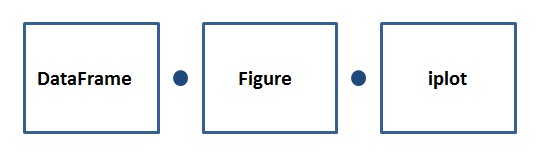• DataFrame：代表pandas的資料框；

• Figure：代表我們上面看到的可繪製圖形，比如bar、box、histogram等等；

• iplot：代表繪製方法，其中有很多引數可以進行配置，調節符合你自己風格的可視化圖形；

````import pandas as pd``import cufflinks as cf``import numpy as np````
````cf.set_config_file(offline=True)````

lines線圖

``cf.datagen.lines(1,500).ta_plot(study='sma',periods=[13,21,55])``

2）figure定義為lines形式，資料為(1,500)；

3）然後再用ta_plot繪製這一組時間序列，引數設置SMA展現三個不同周期的時序分析。

box箱型圖

``cf.datagen.box(20).iplot(kind='box',legend=False)``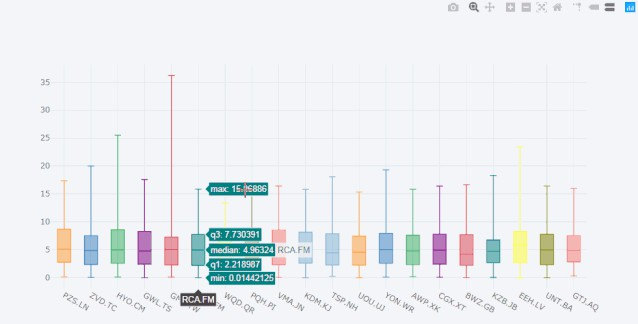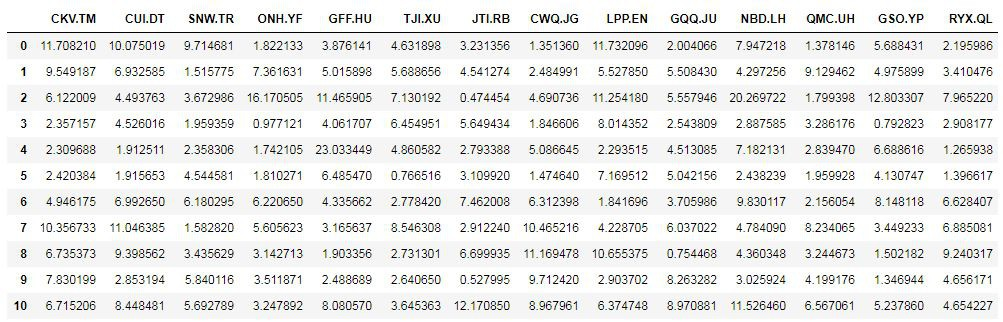histogram直方圖

``cf.datagen.histogram(3).iplot(kind='histogram')``

histogram條形圖

``df=pd.DataFrame(np.random.rand(10, 4), columns=['a', 'b', 'c', 'd'])``df.iplot(kind='bar',barmode='stack')``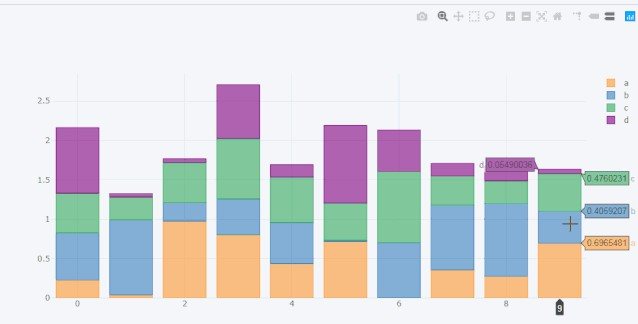scatter散點圖

``df = pd.DataFrame(np.random.rand(50, 4), columns=['a', 'b', 'c', 'd'])``df.iplot(kind='scatter',mode='markers',colors=['orange','teal','blue','yellow'],size=10)``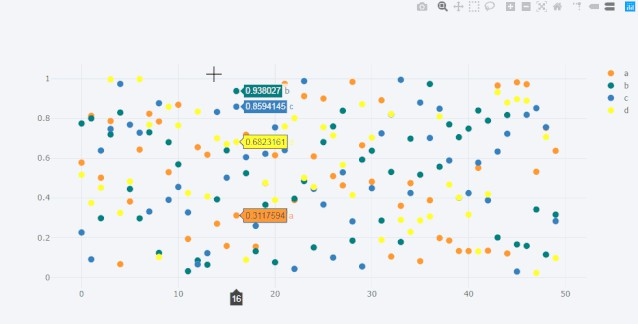bubble氣泡圖

``df.iplot(kind='bubble',x='a',y='b',size='c')``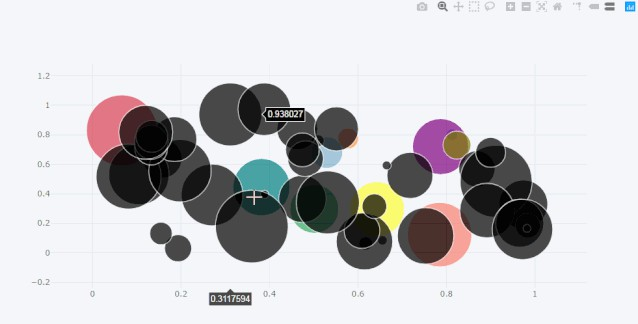scatter matrix 散點矩陣圖

``df = pd.DataFrame(np.random.randn(1000, 4), columns=['a', 'b', 'c', 'd'])``df.scatter_matrix()``

subplots 子圖

``df=cf.datagen.lines(4)``df.iplot(subplots=True,shape=(4,1),shared_xaxes=True,vertical_spacing=.02,fill=True)``

``df.iplot(subplots=True,subplot_titles=True,legend=False)``

``df=cf.datagen.bubble(10,50,mode='stocks')``figs=cf.figures(df,[dict(kind='histogram',keys='x',color='blue'),``                    dict(kind='scatter',mode='markers',x='x',y='y',size=5),``                    dict(kind='scatter',mode='markers',x='x',y='y',size=5,color='teal')],asList=True)``figs.append(cf.datagen.lines(1).figure(bestfit=True,colors=['blue'],bestfit_colors=['pink']))``base_layout=cf.tools.get_base_layout(figs)``sp=cf.subplots(figs,shape=(3,2),base_layout=base_layout,vertical_spacing=.15,horizontal_spacing=.03,``               specs=[[{'rowspan':2},{}],[None,{}],[{'colspan':2},None]],``               subplot_titles=['Histogram','Scatter 1','Scatter 2','Bestfit Line'])``sp['layout'].update(showlegend=False)``cf.iplot(sp)``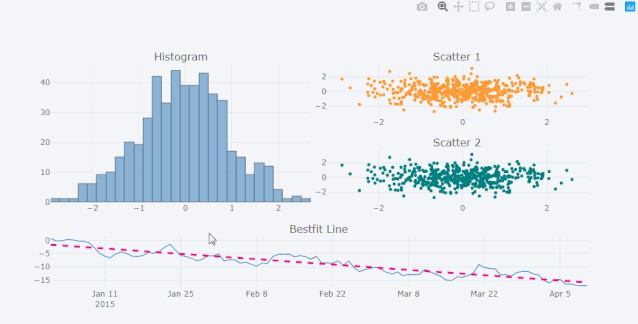shapes 形狀圖

``df=cf.datagen.lines(3,columns=['a','b','c'])``df.iplot(hline=[dict(y=-1,color='blue',width=3),dict(y=1,color='pink',dash='dash')])``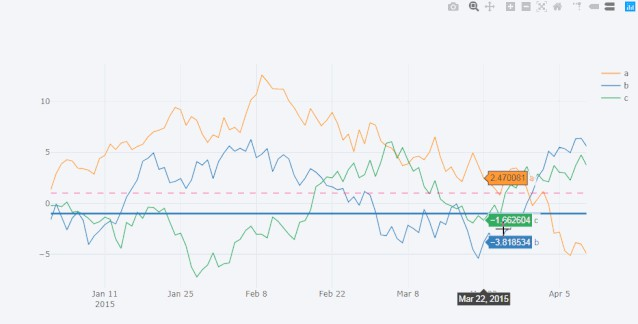``df.iplot(hspan=[(-1,1),(2,5)])``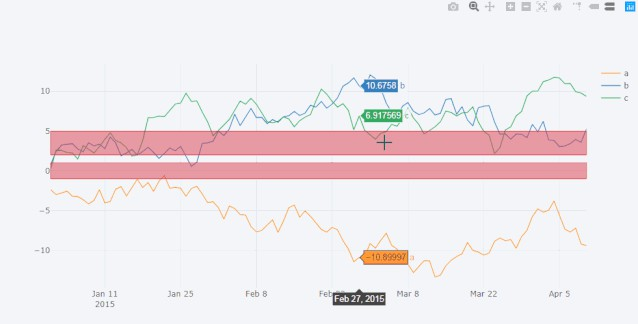``df.iplot(vspan={'x0':'2015-02-15','x1':'2015-03-15','color':'teal','fill':True,'opacity':.4})``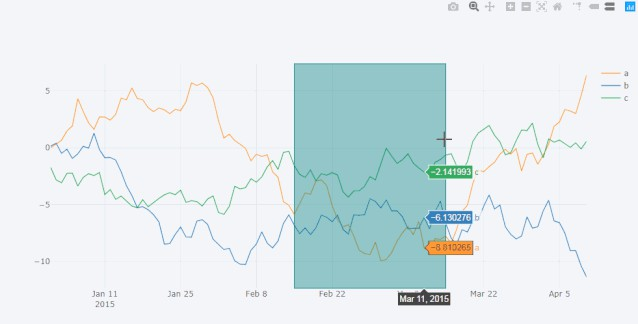``help(df.iplot)``